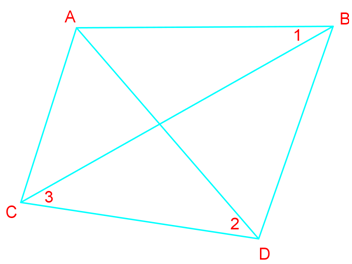# Parallel line proofs

### Parallel line proofs

#### Lessons

• Introduction
Relationships between lines and angles

• 1.
What is the value of x if $\overline {AB}$ $\parallel$ $\overline {CD}$ ?
a)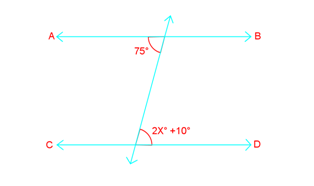b)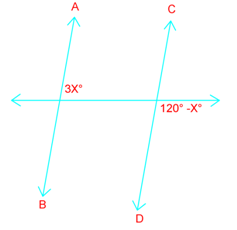c)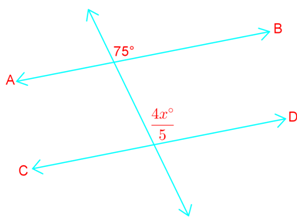• 2.
Determine if enough information is given to prove $p \parallel$ $q$ State the postulates or theorems you use in your answers.
a)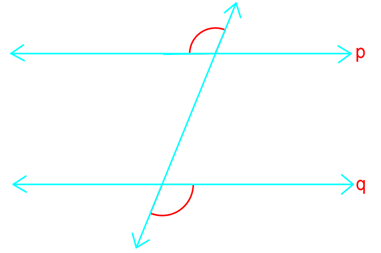b)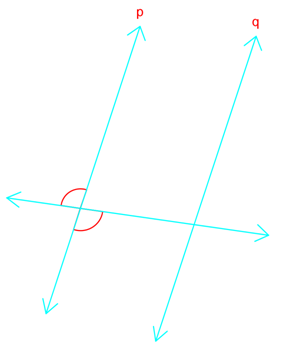c)d)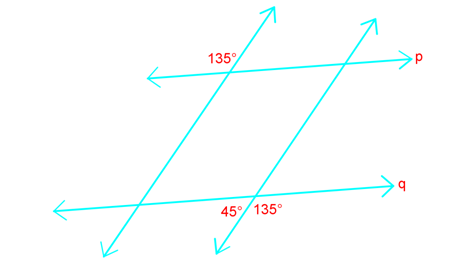• 3.
Prove that if two lines are cut by a transversal so the alternate exterior angles are congruent, then the lines are parallel. (Prove the Alternate Exterior Angles converse)

• 4.
Write a two-column proof.
a)
Given: $m \parallel$ $n$, $\angle 1$ $\cong$$\angle 2$
Prove: $s \parallel$ $t$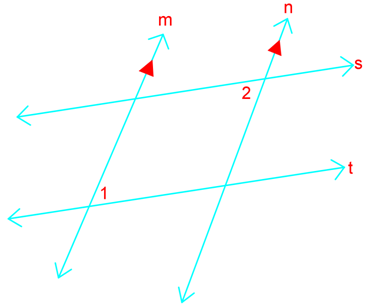b)
Given: $\angle 1$ $\cong$$\angle 2$, $\angle 1$ $\cong$$\angle 3$
Prove: $\overline {AB}$ $\parallel$ $\overline {CD}$